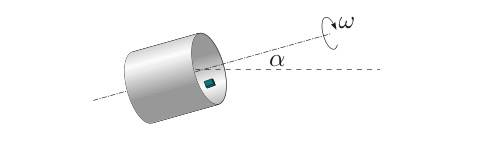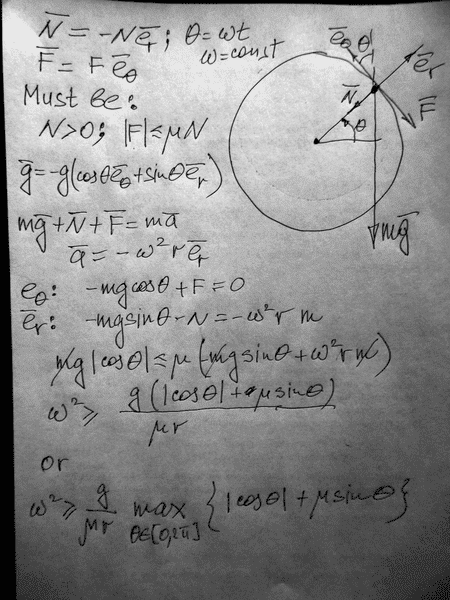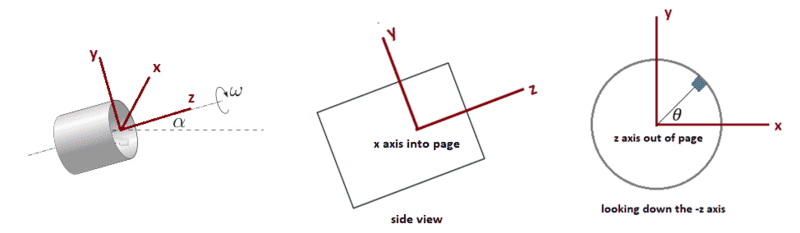# Block in a rotating cylinder

fedecolo

## Homework Statement

A cylinder with radius R spins around its axis with an angular speed ω. On its inner surface there lies a small block; the coefficient of friction between the block and the inner sur- face of the cylinder is μ. Find the values of ω for which the block does not slip (stays still with respect to the cylinder). Consider the cases where (a) the axis of the cylinder is hori- zontal; (b) the axis is inclined by angle α with respect to the horizon.## The Attempt at a Solution

I tried to figure out which are the forces applied to the block in the non inertial frame of the cylinder. There is ##m \vec{g} ##, the centrifugal force ##\vec{F_{c}} = m \omega^2 \vec{R}##, the normal reaction ## \vec{N} ## and the frictional force ## \vec{F_{s}}= \vec{N} \mu ##. If the block is at rest the sum ## m \vec{g} + \vec{F_{c}} + \vec{N} + \vec{F_{s}} = 0 ##.
Considering only case (a) of the problem, I don't know how to work with this equation in a three dimension, because my first solution where that ## \omega = \sqrt{ \frac{\mu g}{R}} ## but I think it's correct in a two-dimensional geometry. Any help or reference to see?

What is the centrifugal force? Is it a force on its own?

fedecolo
No, it's the centripetal force in the reference frame of the rotating cylinder.

Homework Helper
Gold Member
2022 Award
Considering only case (a) of the problem, I don't know how to work with this equation in a three dimension, because my first solution where that ## \omega = \sqrt{ \frac{\mu g}{R}} ## but I think it's correct in a two-dimensional geometry.
That looks right. (I don't see that using the noninertial frame helps here. You get the same equation immediately.)

The 3D version is going to hurt the head.
Consider when the cylinder has rotated θ from the position where the mass is at its highest.
What are the components of the normal unit vector?

By the way, it is not true that ##\vec F_s=\vec N\mu_s##. You only know that on the point of slipping ##|\vec F_s|=|\vec N|\mu_s##.

Considering only case (a) of the problem, I don't know how to work with this equation in a three dimension, because my first solution where that ω=√μgRω=μgR \omega = \sqrt{ \frac{\mu g}{R}} but I think it's correct in a two-dimensional geometry. Any help or reference to see?
What is your work for that? I got a different solution.

•zwierz and TSny
fedecolo
What are the components of the normal unit vector?

I think that ## \vec{N} ##is always directed along the line that connect the mass to the centre of the cylinder, isn't it?

Homework Helper
Gold Member
2022 Award
I think that ## \vec{N} ##is always directed along the line that connect the mass to the centre of the cylinder, isn't it?
Right, but you do not know at what point in the rotation is the greatest risk of slipping. So you need an expression for that vector at a general position. My preference wouldbe to stick with the usual Cartesian coordinates. If you prefer to work with cylindrical here then the challenge, instead, is to represent gravity in those.

zwierz
my result is different as well•fedecolo and Isaac0427
fedecolo
Right, but you do not know at what point in the rotation is the greatest risk of slipping. So you need an expression for that vector at a general position. My preference wouldbe to stick with the usual Cartesian coordinates. If you prefer to work with cylindrical here then the challenge, instead, is to represent gravity in those.

As zwierz did, I have ##N=m \omega^2 R-mg sin \theta ## and ## N= \frac{mg cos \theta}{\mu}## and then ##\mu \geq \frac{g cos \theta}{\omega ^2 R-g sin \theta}## and so ## \omega^2 R \geq g sin \theta ## because the denominator must be > 1 (otherwise the fraction would be > 1 and the block would slide)

•physics_CD
fedecolo
my result is different as well

The final solution must be ## \omega^2 R \geq g\sqrt{1+ \mu ^-2} ##

zwierz
The final solution must be ω2R≥g√1+μ−2
sure

Last edited:
fedecolo
sure

But I don't know how to get it

zwierz
But I don't know how to get it
that is after I have posted the solution ;(

fedecolo
that is after I have posted the solution ;(

The expressions ##\omega^2 R \geq g(cos \theta \mu^-1+ sin \theta) ## and ##\omega^2 R \geq g \sqrt{\mu^-2 +1}## aren't they?

zwierz
Find a maximal value of the function ##f(\theta)=|\cos\theta|+\mu\sin\theta##

fedecolo
Find a maximal value of the function ##f(\theta)=|\cos\theta|+\mu\sin\theta##
Oh you are right, thank you!

The case for part (b) is really not all that different, in terms of how you go about the problem. Now, you just need to find the forces in a third direction; the direction along the length of the cylinder (often denoted by p). Step one is breaking up gravity into its r, θ and p components, in terms of m, g, θ and α. From there, it should be obvious how to continue.

fedecolo
The case for part (b) is really not all that different, in terms of how you go about the problem. Now, you just need to find the forces in a third direction; the direction along the length of the cylinder (often denoted by p). Step one is breaking up gravity into its r, θ and p components, in terms of m, g, θ and α. From there, it should be obvious how to continue.

How can I scompose a three-dimension vector? (I never did something like that) Do you know any reference I can study about it?

my result is different as well
View attachment 203996
Just curious, how did you get the |cosθ|+μsinθ? I got μsinθ-cosθ.

zwierz
Well I have written everything I think about that in #8

Well I have written everything I think about that in #8
Right, but why did you throw in absolute value signs?

EDIT: Never mind, I just realized my mistake.

Last edited:
fedecolo
I'm trying to decompose the 3D vector ##\vec{g}## but I don't know how to figure out it! Any help?

Homework Helper
Gold Member
I'm trying to decompose the 3D vector ##\vec{g}## but I don't know how to figure out it! Any help?
If ##\hat{u}## is a unit vector in some direction, then the component of a vector in the direction of ##\hat{u}## can be found from the dot product of the vector with ##\hat{u}##.

fedecolo
Is it possible that the components are ##\vec{g} (g cos \theta, g sin \theta, g cos \alpha)##?

Homework Helper
Gold Member
Is it possible that the components are ##\vec{g} (g cos \theta, g sin \theta, g cos \alpha)##?

fedecolo

A vector ##\vec{u}## is defined by ##(\vec{u_{x}}, \vec{u_{y}}, \vec{u_{z}})##

Homework Helper
Gold Member
A vector ##\vec{u}## is defined by ##(\vec{u_{x}}, \vec{u_{y}}, \vec{u_{z}})##
Yes. But the values of the components depend on the orientation of the axes. So, you need to describe the orientation of your choice of the x, y, and z axes.

One thing that must be true is that ##g^2 = g_x^2 + g_y^2 + g_z^2##. Is this true for your expression ##\vec{g} = (g cos \theta, g sin \theta, g cos \alpha)##?

Last edited:
A vector ##\vec{u}## is defined by ##(\vec{u_{x}}, \vec{u_{y}}, \vec{u_{z}})##
I'd suggest working in cylindrical coordinates.

fedecolo
Yes. But the values of the components depend on the orientation of the axes. So, you need to describe the orientation of your choice of the x, y, and z.

z is oriented as the axe of rotation of the cylinder if ##\alpha=0##
y is oriented towards the axe of rotation (still if ##\alpha=0##)
x by consequence

fedecolo
I'd suggest working in cylindrical coordinates.

In cylindrical coordinates the axe ##z=\hat{z}##, but I must direct the ##\hat{z}## in the direction of the axe of rotation (inclined by an angle ##\alpha##)?

Homework Helper
Gold Member
z is oriented as the axe of rotation of the cylinder if ##\alpha=0##
y is oriented towards the axe of rotation (still if ##\alpha=0##)
x by consequence
OK, I'm not sure I follow your description of the y axis. Suppose you pick the axes as shown below for ##\alpha \neq 0##. The z axis is the axis of the cylinder and the yz plane is vertical. The x-axis is then horizontal.How would the components of ##\vec{g}## be expressed in this coordinate system?

When the block is at the angle ##\theta## shown, what would be a unit vector in the centripetal direction?

Use these vectors to find the component of ##\vec{g}## that is normal to the surface when the block is in this position.

Last edited:
timetraveller123
hi all I happen to be doing this same problem I am having some problem for part b can I ask it here thaks

Last edited:
Homework Helper
Gold Member
hi all I happen to be doing this same problem I am having some problem for part b can I ask it here thaks
I think that would be OK. The OP, @fedecolo , does not appear to be seeking any more help regarding this problem.

timetraveller123
@TSny
thanks and sorry for the late reply
here is my solution to part a help me check if it is correct it is similar to zweirz's solution

a)
##
f_r = 0 = N - mgsin\theta - mw^2 r\\
N = mgsin\theta +mw^2 r\\
f_\theta = 0 = F - mg cos \theta \\
F = mg cos \theta <= \mu N\\
cos \theta + sin \theta \mu <= \mu \frac{m w^2 r}{ g}\\
##
eyeballing the problem the normal force is clearly least in the first quadrant so the signs are correct
taking derivatives setting zero solving for sin and cos got me
##
\sqrt {\mu ^-2 + 1 } <= \frac{m w^2 r}{g}
##

I tried applying the same procedure for part b I decided to work with cylindrical coordinates with z axis being similar to your and r and theta axis being the usual one along the plane of cylinder

##
g_z = g sin \alpha\\
g_{r,\theta} = g cos \alpha \\
##
so the radial equation is the same as before except g changes
##
f_r = 0 = N + mg cos\alpha sin \theta = m w^2 r\\
N =m w^2 r - mg cos\alpha sin \theta \\
##
now there is two components of friction F_theta and F_z whose comined modulus must be less than \mu N
##
F_\theta = mg cos \alpha cos \theta\\
F_z = mg sin \alpha \\
\sqrt { F_\theta ^2 + F_z ^2}\\
\sqrt {(cos \alpha cos \theta)^2 + sin \alpha ^2} <= \mu(\frac{w^2 r}{g} - cos \alpha sin \theta)\\
cos \alpha(\sqrt {(cos \theta)^2 + tan ^2\alpha } + \mu sin \theta)<= \mu(\frac{w^2 r}{g} )\\
##
after long differentiating I am getting
##
sin \theta = \frac{\mu sec \theta}{\sqrt{\mu^2 + 1}}\\
cos \theta = \sqrt{\frac{1 - \mu^2 tan^2 \alpha }{\mu ^2 +1}}\\
##
plugging back in I get exactly same answer as first part of the problem what am I doing wrong

Homework Helper
Gold Member
I noticed a couple of typographical errors for part (a) which did not affect your subsequent work:
## cos \theta + sin \theta \mu <= \mu \frac{m w^2 r}{ g}##
##\sqrt {\mu ^-2 + 1 } <= \frac{m w^2 r}{g}##
There should not be a factor of ##m## on the right side of the above equations.

Otherwise, the rest of your work looks good except that you need to deal with the following important situation in part (b):

after long differentiating I am getting
##
sin \theta = \frac{\mu sec \theta}{\sqrt{\mu^2 + 1}}\\
cos \theta = \sqrt{\frac{1 - \mu^2 tan^2 \alpha }{\mu ^2 +1}}\\
##
What happens if the quantity inside the square root is negative?

•timetraveller123